Worksheets and No Prep Teaching Resources
Math Worksheets
Measurement Worksheets

# Kindergarten MeasurementWorksheets, Lessons, and Printables

Measurement Mixed Review - Introduction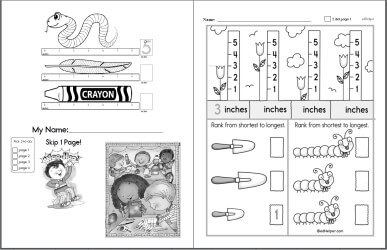Measurement Kindergarten and 1st Grade Workbook

Measuring Length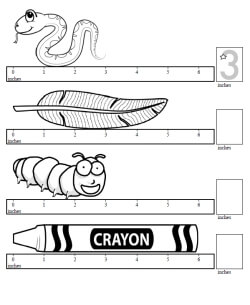Measure length in inches - first answer given Measure length in centimeters - first answer given

Measuring Length of ObjectsMeasure length in inches Measure length in centimeters

Sorting Length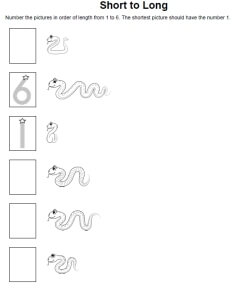Measure length in inches - 1 is shortest, 6 is longest (first and last answers given) Measure length in inches - 1 is longest, 6 is shortest (first and last answers given) Measure length in inches - mix (first and last answers given)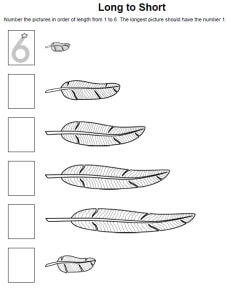Measure length in inches - 1 is shortest, 6 is longest (first answer given) Measure length in inches - 1 is longest, 6 is shortest (first answer given) Measure length in inches - mix (first answer given)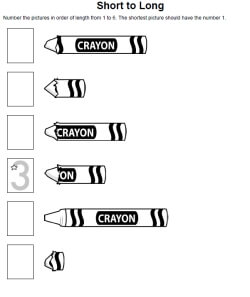Measure length in inches - 1 is shortest, 6 is longest (one answer given) Measure length in inches - 1 is longest, 6 is shortest (one answer given) Measure length in inches - mix (one answer given)